# Using the Math Question Generator

## Introduction

The Learnosity Math Question Generator is a tool for content authors that enables easy creation of Cloze math questions that use dynamic content.

Math Question Generator automates the repetitive aspects of mathematics question authoring, by taking parameter values and then creating a data table containing many variations of the question (and relevant solutions), with validation automatically set up.

Math Question Generator supports all math content currently supported in Learnosity and leverages the same scoring methods as other math question types. However, the Math Question Generator applies the scoring methods for you, depending on the correct answer format you select.

## Usage

To create a Math Question Generator question, add a new item and select the Math Question Generator tile from the math section in the tile view.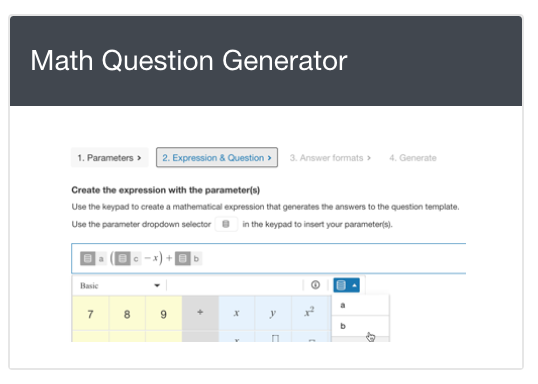Screenshot 1: Math Question Generator in the tile view.

The Math Question Generator authoring interface contains the dynamic math generator wizard, and settings for the following:

• Settings for layout, including final response box size and associated ARIA label
• Settings for text blocks

NOTE: A Math Question Generator question cannot be added to an item with an existingdata table. Additionally, you cannot add two Math Question Generator questions in the same item. Any change to the data table is required to be made within the Math Question Generator wizard.

## Creating the question

When using Math Question Generator for the first time, select the "Create expression" button to launch the wizard. The wizard is a step-by-step progression through four screens to guide content authors through the creation of the question. The four steps are as follows:

1. Create parameters,
2. Create the correct answer and compose the question,
3. Select the correct answer format(s), and
4. Generate the questions.

## 1. Create parameters and set their values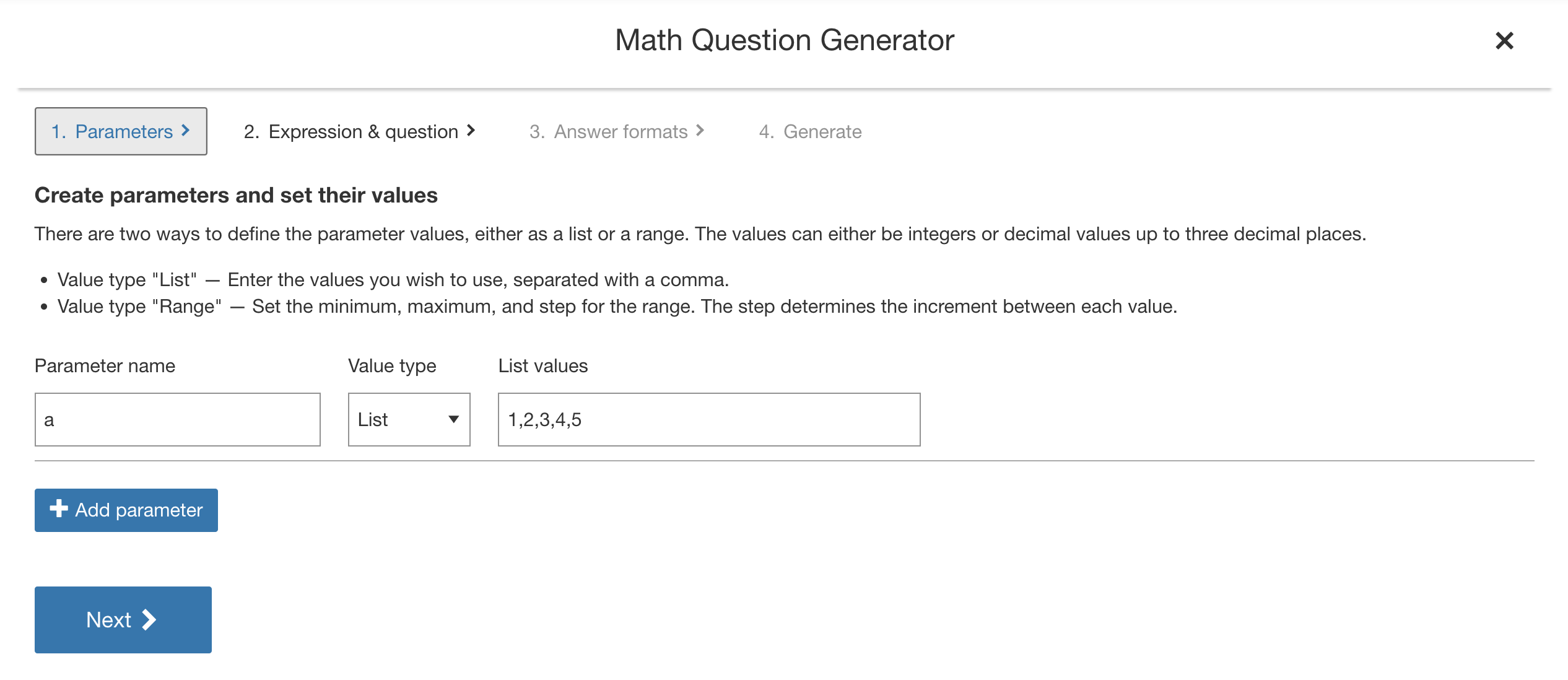Screenshot 2: Creating parameters in Math Question Generator.

In this section, define the parameters that are required for the question. The parameters are the elements that will be exchanged for real values when the item is loaded for the end-user. Parameters can only be alpha characters and can be used within the correct answer and the question stem.

NOTE: There is always a pre-populated parameter, as Math Question Generator requires at least one parameter to operate. When defining parameters, note that Math Question Generator will generate a maximum of 50 question variations in the end.

Once the parameter name has been defined, value settings are applied for the parameters. There are two options for setting parameter values, either as a list or a range. Each option will accept integers or decimal values to three decimal places.

List allows a string of specific, comma-separated values to be defined.

Range allows setting a minimum and maximum for the range of values to be drawn from. This range can be incremented with the step value. The increment will start at the minimum value and finish at the nearest value less than or equal to the maximum. The step will not accept negative values, however does support decimals.

Use the "Add parameter" button to add a new parameter. Parameters can be removed by selecting  "Remove" on the relevant parameter row.

Removing a parameter will remove the associated data in the data table. However, if the parameter has already been added to the Composed question field, the parameter instance in the text will not be removed (it must be manually removed).

Once all parameters and values are set, navigate by clicking "next' or "Expression & question" at the top of the generator wizard.

## 2. Create the correct answer and compose the question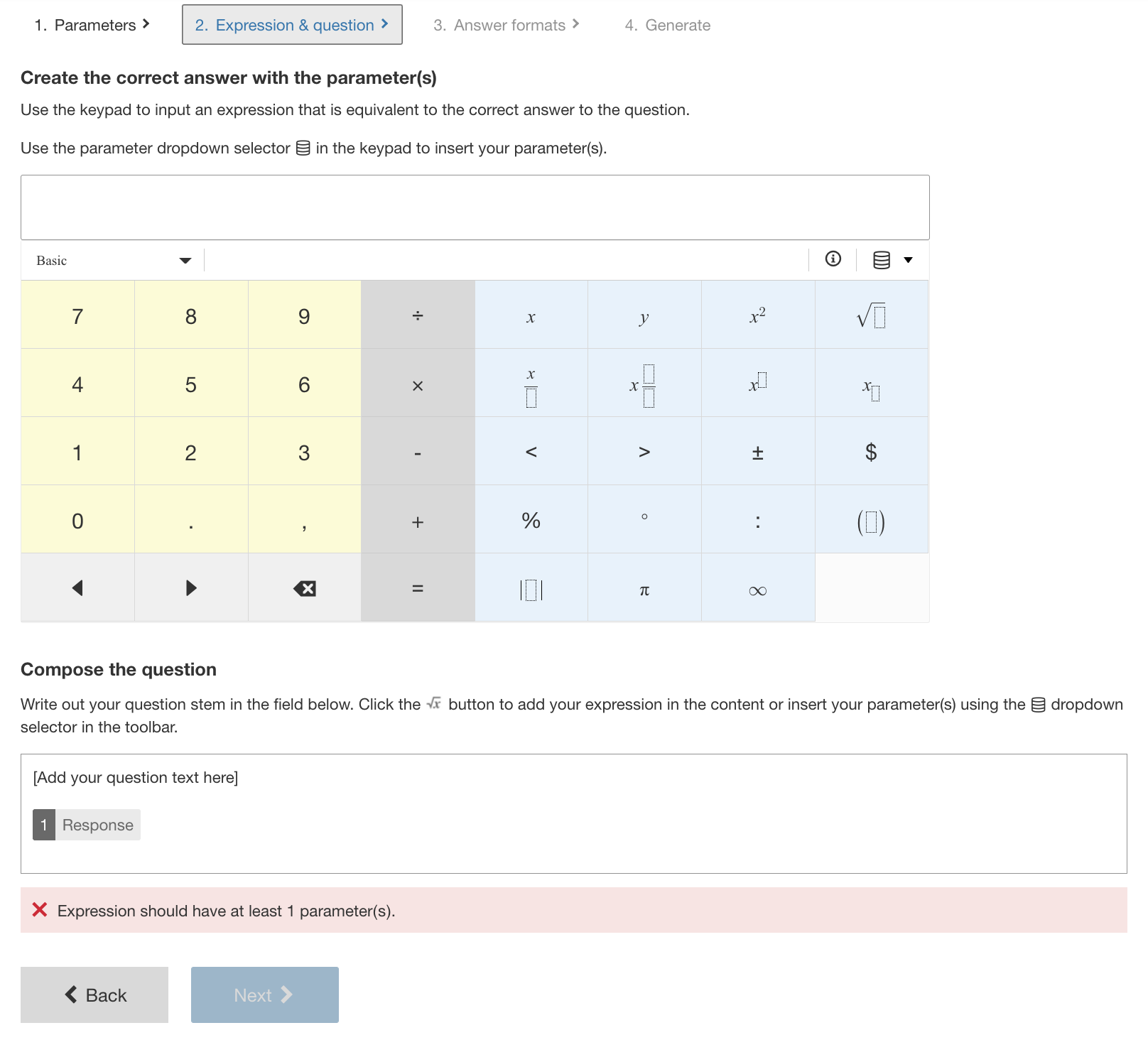Screenshot 3: Create the correct answer and compose the question.

First use the keypad to define the expression that is equivalent to the correct answer to the question. The expression should be in terms of the parameters created previously which are available from the ‘Data'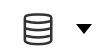dropdown. This expression is the basis for the validation setting (the correct answer) and will be evaluated by Math question generator in the next step.

Next compose the question. The compose question field offers the same rich text options of a regular math question stimulus. The parameters can be added to the question in multiple ways. One option is to add the parameters inline with text, through the ‘data dropdown' buttonwithin the text editor toolbar. The parameters can always be enter as apart of an math expression by using the Math editor in the text editor. Additionally there is the 'Use expression' option to place a copy of the expression entered in the previous field into the question stem, if relevant.

Note: you must ensure that there is a ‘Response’ box included in the question stem. This is the field that the user will enter their answer in. You can only add one ‘response’ area as we do not handle multiple responses with math question generator content.

The default size is 25px square to accommodate its use inline within text. If an alternate size is required this can be adjusted under the Layout section once math question generator content is set and you are back in the question editor. Select preview to see the result as it will be rendered.

## 3. Select the correct answer format(s)

Moving to the next section of the wizard, Math Question Generator will process the expression inputted in the previous correct answer field and display different equivalent mathematical representations. These forms represent the possible correct answers the Learnosity Math engine will accept. Using the check boxes, select the forms of the correct answer that you want to accept.

There any further settings that may be relevant to the form of the correct response. For example, if there are decimals or thousandths in the valid answers, configuration options are displayed to set the accepted forms of the decimal and thousandths separators.

Behind the scenes, Math Question Generator will automatically set the Learnosity math scoring method(s) for the question. When multiple forms are selected, alternate responses are set automatically.

## 4. Generate the questions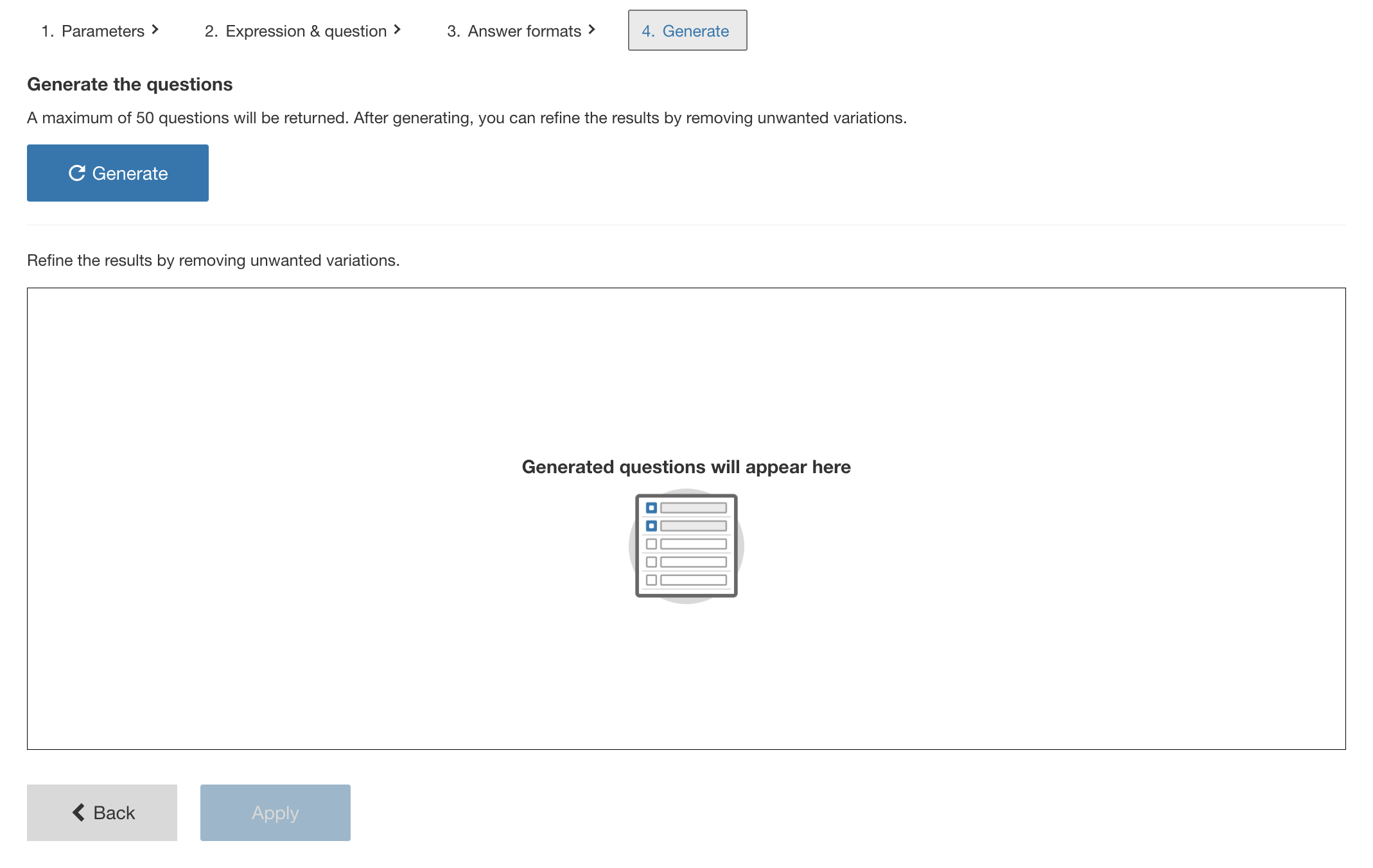The next section of the wizard will allow you to generate the possible variations of the question. This is based on the values for each parameter and the particular form of the correct answer(s) selected. However, only a maximum of 50 variations will be generated.

Select 'generate' to display the populated table of possible parameters and variants of the correct answer forms.

Any unwanted variants can be removed from the final data table. As you scroll through the list of generated results, check those that you want to remove, then select the "Remove" button to remove these from the final data table saved to the item.

To ensure that the data table and the question stem content is committed to the item, it is necessary to select 'Apply'. The data and question content will be saved and returns to the question editor. Here, we can add further settings for the question and preview the results.

## Example

Let’s take the example of asking the learner to expand a polynomial (x-a)(bx-c). The values a, b, and c will become the parameters we set for generation of Math Question Generator content.

As per Step 1, enter these parameters with the following values:
a - set a range from 1 to 5 with a step on 1
b - set a list containing 2, 4, 6
c - set a list containing 1, 3, 5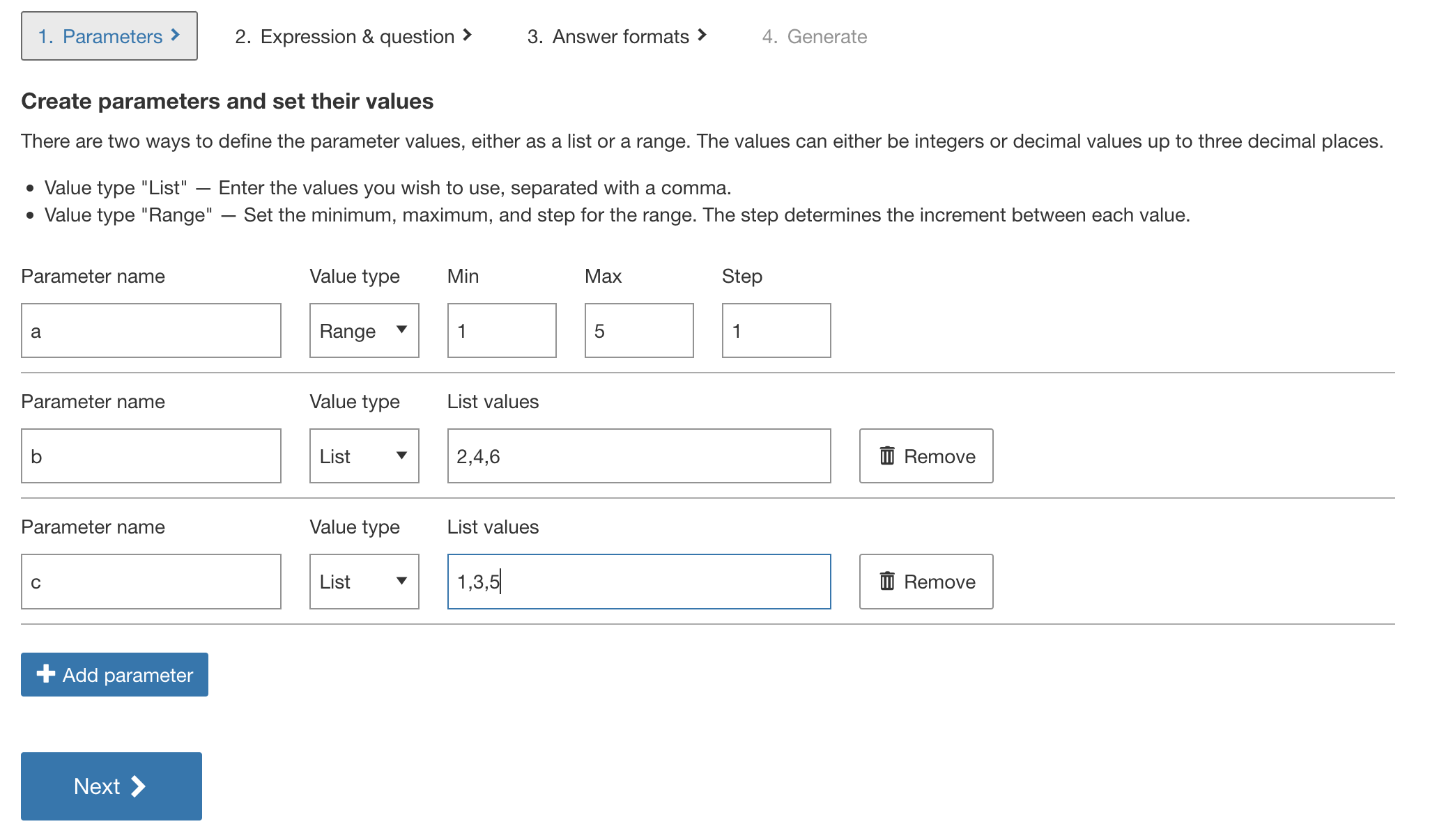Screenshot 5: The parameter for the example.

Now move to the Expression & question section and using the keypad add a mathematical expression that will form the basis for the correct answer to the question. In this example we will enter the factored form of the expression, it should look like this: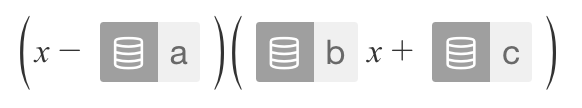Screenshot 6: The expression that generates the correct answer in the example

Moving to the field below the keypad, this is where the question stem is added. We can use the 'Use expression' button to input the polynomial entered above. Below is how this example may be authored.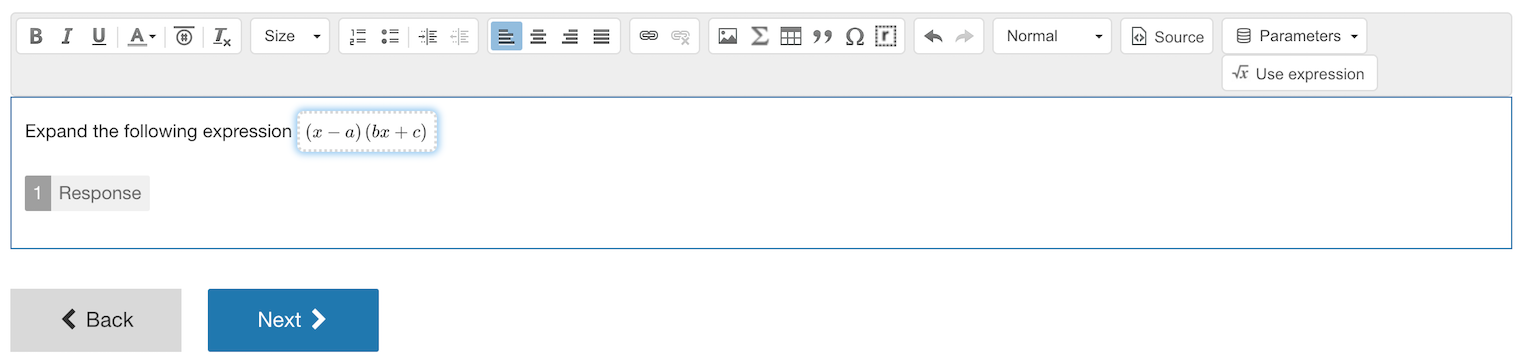Screenshot 7: Authoring your question stem.

Next we move to Step 3, where the various answer formats are generated and displayed for one example parameter set. We will select the Expanded form.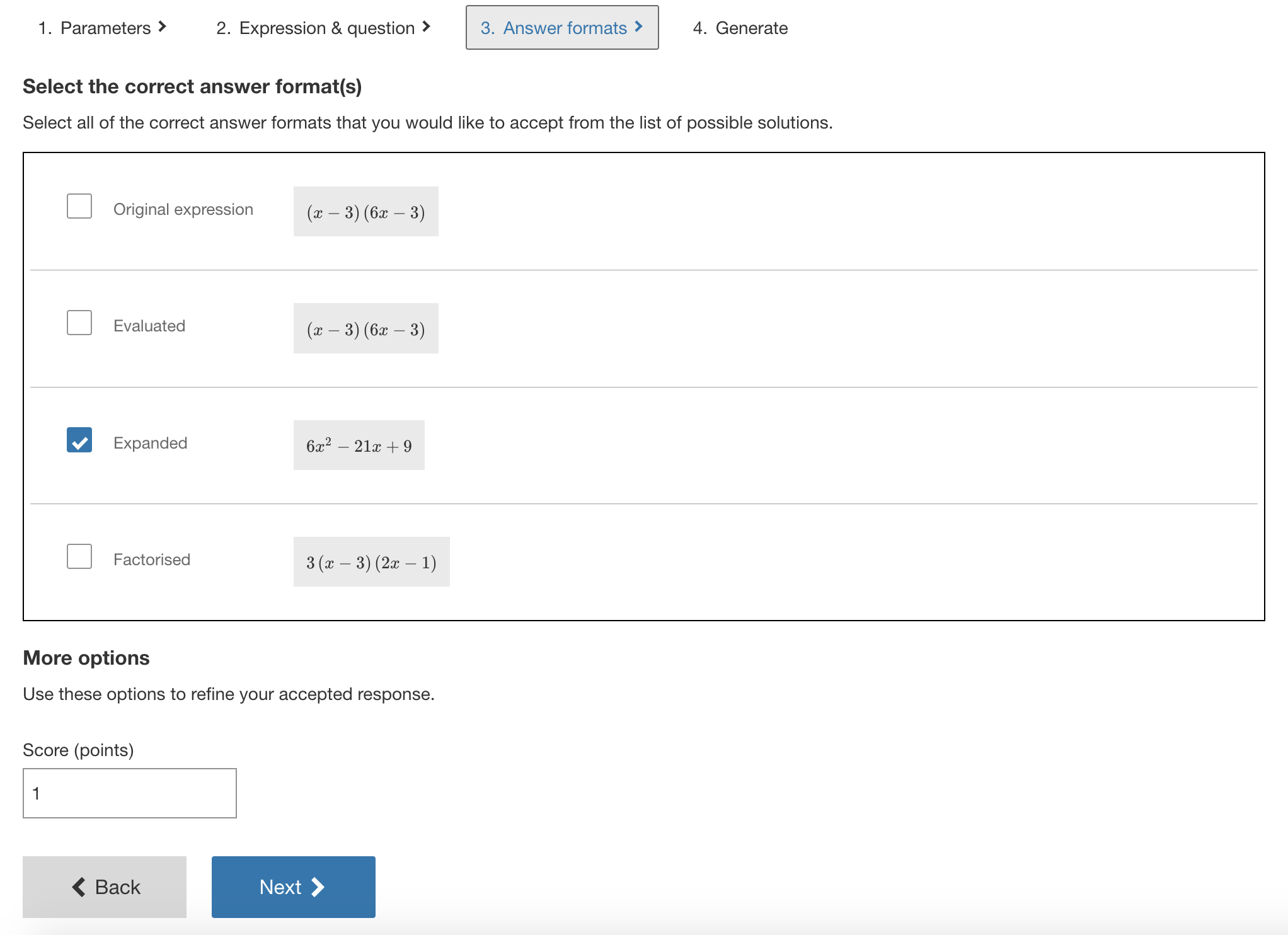Screenshot 8: Selected the "Expanded" correct answer format.

Next we move to Step 4 and generate the variations. Below is an image of the data table showing the parameter combinations and the answer formats. If desired, we could select and remove one of the rows. After we select Apply, we will have created 45 possible variations of this question.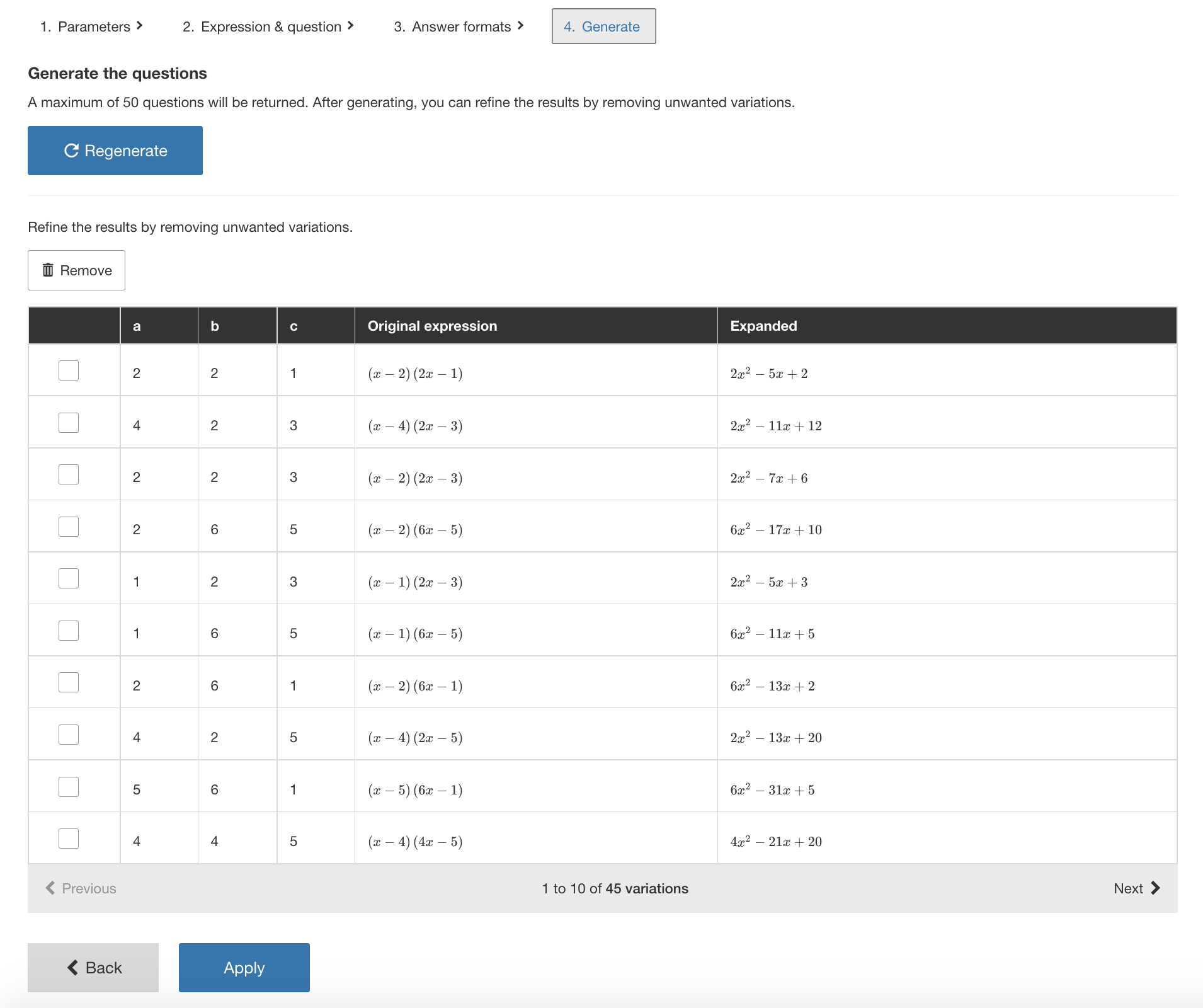Screenshot 9: Generating the variations.